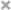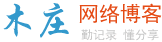# 【c#教程】C# 多态性

C# 多态性

 1234567891011121314151617181920212223242526272829303132 `using` `System;``namespace` `PolymorphismApplication``{``   ``class` `Printdata``   ``{``      ``void` `print(``int` `i)``      ``{``         ``Console.WriteLine(``"Printing int: {0}"``, i );``      ``}` `      ``void` `print(``double` `f)``      ``{``         ``Console.WriteLine(``"Printing float: {0}"` `, f);``      ``}` `      ``void` `print(``string` `s)``      ``{``         ``Console.WriteLine(``"Printing string: {0}"``, s);``      ``}``      ``static` `void` `Main(``string``[] args)``      ``{``         ``Printdata p = ``new` `Printdata();``         ``// 调用 print 来打印整数``         ``p.print(5);``         ``// 调用 print 来打印浮点数``         ``p.print(500.263);``         ``// 调用 print 来打印字符串``         ``p.print(``"Hello C++"``);``         ``Console.ReadKey();``      ``}``   ``}``}`

 123 `Printing ``int``: 5``Printing ``float``: 500.263``Printing ``string``: Hello C++`

C# 允许您使用关键字 abstract 创建抽象类，用于提供接口的部分类的实现。当一个派生类继承自该抽象类时，实现即完成。抽象类包含抽象方法，抽象方法可被派生类实现。派生类具有更专业的功能。

 12345678910111213141516171819202122232425262728293031323334 `using` `System;``namespace` `PolymorphismApplication``{``   ``abstract` `class` `Shape``   ``{``      ``public` `abstract` `int` `area();``   ``}``   ``class` `Rectangle:  Shape``   ``{``      ``private` `int` `length;``      ``private` `int` `width;``      ``public` `Rectangle( ``int` `a=0, ``int` `b=0)``      ``{``         ``length = a;``         ``width = b;``      ``}``      ``public` `override` `int` `area ()``      ``{ ``         ``Console.WriteLine(``"Rectangle 类的面积："``);``         ``return` `(width * length); ``      ``}``   ``}` `   ``class` `RectangleTester``   ``{``      ``static` `void` `Main(``string``[] args)``      ``{``         ``Rectangle r = ``new` `Rectangle(10, 7);``         ``double` `a = r.area();``         ``Console.WriteLine(``"面积： {0}"``,a);``         ``Console.ReadKey();``      ``}``   ``}``}`

 12 `Rectangle 类的面积：``面积： 70`

 12345678910111213141516171819202122232425262728293031323334353637383940414243444546474849505152535455565758596061626364 `using` `System;``namespace` `PolymorphismApplication``{``   ``class` `Shape ``   ``{``      ``protected` `int` `width, height;``      ``public` `Shape( ``int` `a=0, ``int` `b=0)``      ``{``         ``width = a;``         ``height = b;``      ``}``      ``public` `virtual` `int` `area()``      ``{``         ``Console.WriteLine(``"父类的面积："``);``         ``return` `0;``      ``}``   ``}``   ``class` `Rectangle: Shape``   ``{``      ``public` `Rectangle( ``int` `a=0, ``int` `b=0): ``base``(a, b)``      ``{` `      ``}``      ``public` `override` `int` `area ()``      ``{``         ``Console.WriteLine(``"Rectangle 类的面积："``);``         ``return` `(width * height); ``      ``}``   ``}``   ``class` `Triangle: Shape``   ``{``      ``public` `Triangle(``int` `a = 0, ``int` `b = 0): ``base``(a, b)``      ``{``      ` `      ``}``      ``public` `override` `int` `area()``      ``{``         ``Console.WriteLine(``"Triangle 类的面积："``);``         ``return` `(width * height / 2); ``      ``}``   ``}``   ``class` `Caller``   ``{``      ``public` `void` `CallArea(Shape sh)``      ``{``         ``int` `a;``         ``a = sh.area();``         ``Console.WriteLine(``"面积： {0}"``, a);``      ``}``   ``}  ``   ``class` `Tester``   ``{``      ` `      ``static` `void` `Main(``string``[] args)``      ``{``         ``Caller c = ``new` `Caller();``         ``Rectangle r = ``new` `Rectangle(10, 7);``         ``Triangle t = ``new` `Triangle(10, 5);``         ``c.CallArea(r);``         ``c.CallArea(t);``         ``Console.ReadKey();``      ``}``   ``}``}`

 1234 `Rectangle 类的面积：``面积：70``Triangle 类的面积：``面积：25`

C#开发之winform（公共控件）

C#如何连接数据库？oledbconnection与sqlconnection的区别

C# windowsapi应用之flashwindowex -实现窗口闪烁的方法详解

C#教程】C# 枚举（enum）

C# socket实现简单控制台案例

C#如何使用浏览按钮获得文件路径和文件夹路径的实现方法

C#灵活使用类的方法分享### 评论

• 欢迎访问木庄网络博客
• 可复制：代码框内的文字。
• 方法：Ctrl+C。